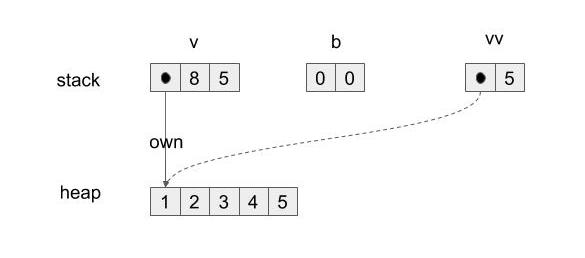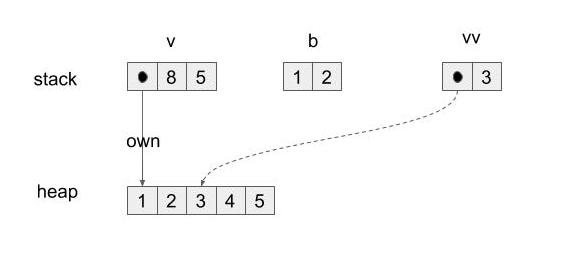# Learn fat pointer from Read trait

Read trait is a trait that abstracts reading something from somewhere and the core method is the `read`. Let's see the type.

```pub trait Read {
#[stable(feature = "rust1", since = "1.0.0")]
fn read(&mut self, buf: &mut [u8]) -> Result<usize>;
```

The implementation of `&[u8]` teaches us a lot.

```impl<'a> Read for &'a [u8] {
#[inline]
fn read(&mut self, buf: &mut [u8]) -> io::Result<usize> {
let amt = cmp::min(buf.len(), self.len());
let (a, b) = self.split_at(amt);

// First check if the amount of bytes we want to read is small:
// `copy_from_slice` will generally expand to a call to `memcpy`, and
// for a single byte the overhead is significant.
if amt == 1 {
buf = a;
} else {
buf[..amt].copy_from_slice(a);
}

*self = b;
Ok(amt)
}
```

Since self is of type `&[u8]` the type signature of read is now `read(&mut &[u8], &mut [u8])` where the first argument is a buffer we read from and the second argument is the buffer we write into. The interesting stuff here is that the first one has an ampersand while the second doesn't. What is the difference between these two?

Let's learn from an actual code that takes `&[u8]` slice from `Vec<u8>`.

```fn main() {
let mut v: Vec<u8> = vec![0,1,2,3,4];
let mut b = [0;2];
let mut vv: &[u8] = &v[..];
vv.read(&mut b).unwrap();
}
```

The memory placement will be like this:)

`Vec<u8>` data is on the heap and the ownership pointer with (pointer, capacity, length) is on the stack. The slice is a fat pointer to the data. The buffer of the second argument is on the stack because it is a fixed size array.

Then the each meaning of the arguments will be:

• 1st argument`&mut &[u8]`: it can mutate the fat pointer itself.
• 2nd argument`&mut [u8]`: it can mutate the data on the stack.

Getting back to the `&[u8]` implementation we see that it computes the copy length `amt` and separates the 1st buffer into what to be consumed and what will be remained using `split_at` function. `split_at` is defined in slice type `[T]` with the type signature

```pub fn split_at(&self, mid: usize) -> (&[T], &[T])
```

If T is u8 then it simply means it separates a single `&[u8]` into two `&[u8]`s.

Then it overwrites the fat pointer of remaining part to self:

```*self = b
```

Now we understand the 1st argument needs to be `&mut` because of this.

After everything done, the memory placement would be like this: